# Image filtering assignment help sample

Image filtering helps us obtain sharper, clearer images. If you are struggling with an assignment from this concept, feel free to get in touch with our experts. We also provide online tutoring on this topic.

## Image Filtering

STRING PROCESSING IN C
The name of your program file should be analysis.c
The results should be in the form of five tables:
Table 1a: alphabet versus occurrences
Table 1b: word lengths versus occurrences (the example table given on page 348)
Table 1c: words versus occurrences
Table 1d: words versus occurrences (sorted by words)
Table 1e: words versus occurrences (sorted by occurrence)
Note: Your program should read input from a text file and should be able to write output to another text file, depending onuser’s input. If the user does not specify any output file, the results will be displayed on the screen.
Example command line input after appropriate compilation:
\$> analysis inText.txt outText.txt /*input from inText.txt, output to outText.txt
\$> analysis inText.txt /*input from inText.txt, output to the screen

2. IMAGE PROCESSING IN C (IMAGE EDGE DETECTION)
Note: this particular assignment will form the basis for the OS-assignment, which will involve imagemanipulation using many processes or threads. Thus, an understanding of thisproblem will significantly reduce the time you will need in the OS assignment.
Given an m×mimage A, the required task is to detect edges in the image. This type of operation is a standard practice in imageprocessing and computer vision, and is a required step in tasks, such as automated object recognition by a robot or by amachine, in shape retrieval and classification, and in biometrics. It is also performed during the stage of image and videocompression, for edge-based compression schemes. For simplicity, we assume that the input image is always square. The required edge detection is performed using a 2D filtering mask as follows.
Step 1: Filtering
Define a 2D mask as an n×nmatrix. For now we assume n is a small odd number, example n=3, 5, or 7. For each n×nblockwe compute the product of the values in the mask with the corresponding position in the small block. We replace the value atthe center of the small block from the image with the sum of the products. Specifically, if I(x,y) is the pixel value at position(x,y) in the image I, and E(x, y) is resulting edge response after the filtering using the mask w. The edge response is obtained as: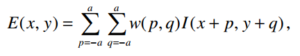wherea=(n-1)/2 .
The filtering is performed using a horizontal mask (wh) and a vertical mask (wv).
Each mask produces a component image, which are then combined to obtain the final edge response image.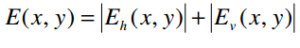For this assignment, we perform simple Sobel edge detection, with the masks defined by the following matrices: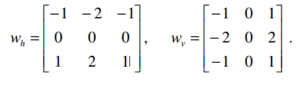Step 2: Binary Image
The edge response image is then binarized using a threshold to obtain a binary edge image. To determine a threshold, we compute the mean (µ) and standard deviation (σ) of the edge responses. We define the threshold simply as follows: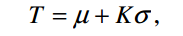where K is a constant. We set K=1 for now. The binary image is then defined as :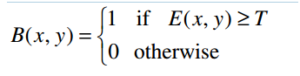The figure below shows an example for both vertical, horizontal, and combined edges, without the binary image.

Your program should produce the respective edge response images (as above), and also the corresponding binary edge images.
The main function will thus do the following:
i. Read the input image (imageIn.ppm)
ii. Select smaller overlapping subimages (or blocks) each of size n×n
iii. Send each image block to another function, (say an image-analysis function), which then analyzes the image andreturns the results.
iv. Wait for the called function to return the results
v. Arrange the returned results to form the edge response images.
vi. Compute the final respective binary images for the horizontal, vertical, and combined edge responses.
vii. Write the resulting images to disk as output files
viii. Calculate and printout the overall mean and standard deviation for the original image, and for each edge responseimage.
The actual task that the image-analysis function will perform is to compute the horizontal, vertical and combined edgeresponses using the image block passed to it by the client. That is, the function will:
i. Receive the input image block from the calling function
ii. Perform that image analysis task
iii. Send back the results to the client
Note: given a collection of numbers, x1, x1, x3,…,xn the mean and standard deviation are defined respectively as: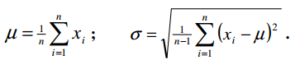The Input Data
The input data will be an image in ppm (portable pixel format) to be provided by the user. Also provided are two functions, one to read images in ppm format, and the other to create a ppmimage from a given data. These functions are in the image library: iplib2New.c, available in imageInfo directory. A sampleprogram for using the image library is given in the file: ip03mainSample.c. You are not required to create your own programfor reading or writing image files. All you need is to understand the interface to the functions – i.e. how to pass parametersto the functions (essentially, the function prototypes). Assume that images are only gray-level images. Example Command Line
ourShell\$>imEdgeimageIn.ppmoutEh.ppmoutEv.ppmoutE.ppmoutBh.ppmoutBv.ppmoutB.ppm

Your program should be in C, and should be able to run on a Unix/Linux platform. The program should be called imEdge.c .

ip03mainSample.c

#include

#include

#include “iplib2New.c”

#define rr 200

#define cc 225

// typedef unsigned char *image_ptr;

image_ptrread_pnm(char *filename, int *rows, int *cols, int *type);

intgetnum(FILE *fp);

voidwrite_pnm(image_ptrptr, char *filename, int rows, intcols, int type);

int ROWS, COLS, TYPE;

int main(intargc, char **argv)

{

image_ptrimagePtr, imagePtr2;

unsigned char image2

[rr]

[cc]

; /* space for output image */

image_ptrimageNegPtr;

int rows, cols, type;

int i=0, j=0, value=0, count=0;

inttp;

doubleave=0;

charaveStr, *aveStrEnd;

/* check inputs */

if (argc != 4)

{

printf(“wrong inputs: use %s infile out1 out2 \n”, argv);

return 0;

}

/* first read-in the image */

imagePtr = read_pnm(argv, &rows, &cols, &type);

printf(“rows=%d, cols=%d, type=%d \n”, rows, cols, type);

/* printf(“rows=%d, cols=%d, type=%d \n”, ROWS, COLS, TYPE); */

/* print out some greylevel pixel values */

value=0;

for (i=0; i< 10; i++)

{ value = value + imagePtr[i];

ave=(double) value/(i+1);

/* aveStr = lltostr((long long) value, aveStrEnd); */

sprintf(aveStr, “%f”, ave);

printf(“i=%d, pixel value=%d, sum=%d, ave=%s\n”,i, imagePtr[i], value, aveStr);

}

/*

/* now write a small image subblock to a file – to argv

/* simply use the first rr x cc pixel block in the input image

*/

tp = 5; /* type==5 */

count=0;

for (i=0; i

for (j=0; j< cc; j++)

{

image2[i][j]=imagePtr[i*cols + j];

}

/* image2 now contains our rr x cc sub-image block */

imagePtr2=(image_ptr) image2;

//…

// you can do calculations on the image pixels here

//…

printf(“\n Now writing to image file … \n”);

printf(“rows=%d, cols=%d, type=%d \n”, rr, cc, tp);

write_pnm(imagePtr2, argv, rr, cc, tp);

/* image negatives */

imageNegPtr=(image_ptr) malloc(rows*cols*(sizeof(unsigned char)) );

// … codes for image negative here

printf(“\n Now writing negative image file … \n”);

printf(“rows=%d, cols=%d, type=%d \n”, rows, cols, type);

write_pnm(imageNegPtr, argv, rows, cols, type);

return 0;

ip03mainSample_1.c

#include

#include

#include “iplib2New.c”

#define rr 200

#define cc 225

// typedef unsigned char *image_ptr;

image_ptrread_pnm(char *filename, int *rows, int *cols, int *type);

intgetnum(FILE *fp);

voidwrite_pnm(image_ptrptr, char *filename, int rows, intcols, int type);

int ROWS, COLS, TYPE;

int main(intargc, char **argv)

{

image_ptrimagePtr, imagePtr2;

unsigned char image2

[rr]

[cc]

; /* space for output image */

image_ptrimageNegPtr;

int rows, cols, type;

int i=0, j=0, value=0, count=0;

inttp;

doubleave=0;

charaveStr, *aveStrEnd;

/* check inputs */

if (argc != 4)

{

printf(“wrong inputs: use %s infile out1 out2 \n”, argv);

return 0;

}

/* first read-in the image */

imagePtr = read_pnm(argv, &rows, &cols, &type);

printf(“rows=%d, cols=%d, type=%d \n”, rows, cols, type);

/* printf(“rows=%d, cols=%d, type=%d \n”, ROWS, COLS, TYPE); */

/* print out some greylevel pixel values */

value=0;

for (i=0; i< 10; i++)

{ value = value + imagePtr[i];

ave=(double) value/(i+1);

/* aveStr = lltostr((long long) value, aveStrEnd); */

sprintf(aveStr, “%f”, ave);

printf(“i=%d, pixel value=%d, sum=%d, ave=%s\n”,i, imagePtr[i], value, aveStr);

}

/*

/* now write a small image subblock to a file – to argv

/* simply use the first rr x cc pixel block in the input image

*/

tp = 5; /* type==5 */

count=0;

for (i=0; i

for (j=0; j< cc; j++)

{

image2[i][j]=imagePtr[i*cols + j];

}

/* image2 now contains our rr x cc sub-image block */

imagePtr2=(image_ptr) image2;

//…

// you can do calculations on the image pixels here

//…

printf(“\n Now writing to image file … \n”);

printf(“rows=%d, cols=%d, type=%d \n”, rr, cc, tp);

write_pnm(imagePtr2, argv, rr, cc, tp);

/* image negatives */

imageNegPtr=(image_ptr) malloc(rows*cols*(sizeof(unsigned char)) );

// … codes for image negative here

printf(“\n Now writing negative image file … \n”);

printf(“rows=%d, cols=%d, type=%d \n”, rows, cols, type);

write_pnm(imageNegPtr, argv, rows, cols, type);

return 0;

}

iplib2New.c

#include

#include

/* file types*/

#define PBM 4

#define PGM 5

#define PPM 6

typedef unsigned char *image_ptr;

image_ptrread_pnm(char *filename, int *rows, int *cols, int *type);

intgetnum(FILE *fp);

voidwrite_pnm(image_ptrptr, char *filename, int rows, intcols, int type);

int ROWS, COLS, TYPE;

/***************************************************************************

* *

* Desc: reads a portable bitmap file *

* *

* Params: filename – name of image file to read *

* rows – number of rows in the image *

* cols – number of columns in the image *

* type – file type *

* *

* Returns: pointer to the image just read into memory *

***************************************************************************/

image_ptrread_pnm(char *filename, int *rows, int *cols, int *type)

{

int i; /* index variable */

introw_size; /* size of image row in bytes */

intmaxval; /* maximum value of pixel */

FILE *fp; /* input file pointer */

intfirstchar, secchar; /* first 2 characters in the input file */

image_ptrptr; /* pointer to image buffer */

unsigned long offset; /* offset into image buffer */

unsigned long total_size; /* size of image in bytes */

unsigned long total_bytes; /* number of total bytes written to file */

float scale; /* number of bytes per pixel */

/* open input file */

if((fp = fopen(filename, “rb”)) == NULL)

{

printf(“Unable to open %s for reading\n”,filename);

exit(1);

}

firstchar = getc(fp);

secchar = getc(fp);

printf(“second char %d \n”, secchar);

if(firstchar != ‘P’)

{

printf(“Sorry… This is not a PPM file!\n”);

exit(1);

}

*cols = getnum(fp);

*rows = getnum(fp);

*type = secchar – ‘0’;

ROWS = *rows; COLS = *cols; TYPE = *type;

switch(secchar)

{

case ‘4’: /* PBM */

scale = 0.125;

maxval = 1;

break;

case ‘5’: /* PGM */

scale = 1.0;

maxval = getnum(fp);

break;

case ‘6’: /* PPM */

scale = 3.0;

maxval = getnum(fp);

break;

default : /* Error */

printf(“read_pnm: This is not a Portable bitmap RAWBITS file\n”);

exit(1);

break;

}

row_size = (*cols) * scale;

total_size = (unsigned long) (*rows) * row_size;

ptr = (image_ptr) malloc(total_size);

if(ptr == NULL)

{

printf(“Unable to malloc %lu bytes\n”,total_size);

exit(1);

}

total_bytes=0;

offset = 0;

for(i=0; i<(*rows); i++)

{

offset += row_size;

}

if(total_size != total_bytes)

{

total_size, total_bytes);

exit(1);

}

fclose(fp);

returnptr;

}

/***************************************************************************

* Func: getnum *

* *

* Desc: reads an ASCII number from a portable bitmap file header *

* *

* Param: fp – pointer to file being read *

* *

* Returns: the number read *

***************************************************************************/

intgetnum(FILE *fp)

{

char c; /* character read in from file */

int i; /* number accumulated and returned */

do

{

c = getc(fp);

}

while((c==’ ‘) || (c==’\t’) || (c==’\n’) || (c==’\r’));

if((c<‘0′) || (c>’9’))

if(c == ‘#’) /* chew off comments */

{

while(c == ‘#’)

{

while(c != ‘\n’)

c = getc(fp);

c = getc(fp);

}

}

else

{

printf(“Garbage in ASCII fields\n”);

exit(1);

}

i=0;

do

{

i=i*10+(c-‘0′); /* convert ASCII to int */

c = getc(fp);

}

while((c>=’0′) && (c<=’9’));

return i;

}

/***************************************************************************

* Func: write_pnm *

* *

* Desc: writes out a portable bitmap file *

* *

* Params: ptr – pointer to image in memory *

* filename _ name of file to write image to *

* rows – number of rows in the image *

* cols – number of columns in the image *

* magic_number – number that defines what type of file it is *

* *

* Returns: nothing *

***************************************************************************/

voidwrite_pnm(image_ptrptr, char *filename, int rows,

intcols, intmagic_number)

{

FILE *fp; /* file pointer for output file */

long offset; /* current offset into image buffer */

longtotal_bytes; /* number of bytes written to output file */

longtotal_size; /* size of image buffer */

introw_size; /* size of row in bytes */

int i; /* index variable */

float scale; /* number of bytes per image pixel */

switch(magic_number)

{

case 4: /* PBM */

scale = 0.125;

break;

case 5: /* PGM */

scale = 1.0;

break;

case 6: /* PPM */

scale = 3.0;

break;

default : /* Error */

printf(“write_pnm: This is not a Portable bitmap RAWBITS file\n”);

exit(1);

break;

}

/* open new output file */

if((fp=fopen(filename, “wb”)) == NULL)

{

printf(“Unable to open %s for output\n”,filename);

exit(1);

}

/* print out the portable bitmap header */

fprintf(fp, “P%d\n%d %d\n”, magic_number, cols, rows);

if(magic_number != 4)

fprintf(fp, “255\n”);

row_size = cols * scale;

total_size = (long) row_size *rows;

offset = 0;

total_bytes = 0;

for(i=0; i

{

total_bytes += fwrite(ptr+offset, 1, row_size, fp);

offset += row_size;

}

if(total_bytes != total_size)

printf(“Tried to write %ld bytes…Only wrote %ld\n”,

total_size, total_bytes);

fclose(fp);

Solution

analysis.c

#include

#include

#include

#include

#include

/* Maximum word length. Longer words will be skipped and error message will be

* issued. */

#define MAX_WORD_LENGTH 32

/* Structure for storing the number of occurences of a word. */

typedefstruct Word {

char *word;

int count;

} Word;

/* Word counts. */

Word *stat_words;

/* Number of words in the stat_words array. */

intstat_nwords;

/* Letter count. */

intstat_alphabet;

/* Word length count. */

intstat_lengths[MAX_WORD_LENGTH];

/* Comparison function. Compares pointers to two words. Returns an integer less

* than, equal to, or greater than zero if the first word is considered to be

* respectively less than, equal to, or greater than the second. */

intword_compar(const void *a, const void *b) {

returnstrcmp(((Word *)a)->word, ((Word *)b)->word);

}

/* Comparison function. Compares pointers to two words. Returns an integer less

* than, equal to, or greater than zero if the count of the first word is

* considered to be respectively greater, equal to, or less than than the

* count of the second. */

intcount_compar(const void *a, const void *b) {

return ((Word *)b)->count – ((Word *)a)->count;

}

intis_word_char(int c) {

return (c >= ‘a’ && c <= ‘z’) || (c >= ‘A’ && c <= ‘Z’) || c == ‘\”;

}

/* Print table 1a: alphabet versus occurrences */

void print_table_1a(FILE *output) {

/* Lowercase character. Used in a loop. */

intlc;

fprintf(output, “Table 1a: alphabet versus occurrences\n\n”);

fprintf(output, “————————————————\n”);

fprintf(output, “| %13s | %13s | %10s |\n”, “lower case”, “upper case”,

“total”);

fprintf(output, “————————————————\n”);

for (lc = ‘a’; lc<= ‘z’; ++lc) {

intuc = lc – ‘a’ + ‘A’;

fprintf(output, “| %c | %10d | %c | %10d | %10d |\n”, lc, stat_alphabet[lc],

uc, stat_alphabet[uc], stat_alphabet[lc] + stat_alphabet[uc]);

}

fprintf(output, “————————————————“);

}

/* Print table 1b: word lengths versus occurrences (the example table given on

* page 348) */

void print_table_1b(FILE *output) {

int length;

fprintf(output, “\n\nTable 1b: word lengths versus occurrences\n\n”);

fprintf(output, “———————–\n”);

fprintf(output, “| length | count |\n”);

fprintf(output, “———————–\n”);

for (length = 1; length < MAX_WORD_LENGTH; ++length) {

fprintf(output, “| %6d | %10d |\n”, length, stat_lengths[length]);

}

fprintf(output, “———————–\n”);

}

/* Print table 1c: words versus occurrences */

void print_table_1c(FILE *output) {

int i;

fprintf(output, “\n\nTable 1c: words versus occurrences\n\n”);

fprintf(output, “————————————-\n”);

fprintf(output, “| word | count |\n”);

fprintf(output, “————————————-\n”);

for (i = 0; i

fprintf(output, “| %20s | %10d |\n”, stat_words[i].word,

stat_words[i].count);

}

fprintf(output, “————————————-\n”);

}

/* Print table 1d: words versus occurrences (sorted by words) */

void print_table_1d(FILE *output) {

int i;

qsort(stat_words, stat_nwords, sizeof(Word), word_compar);

fprintf(output,

“\n\nTable 1d: words versus occurrences (sorted by words)\n\n”);

fprintf(output, “————————————-\n”);

fprintf(output, “| word | count |\n”);

fprintf(output, “————————————-\n”);

for (i = 0; i

fprintf(output, “| %20s | %10d |\n”, stat_words[i].word,

stat_words[i].count);

}

fprintf(output, “————————————-\n”);

}

/* Print table 1e: words versus occurrences (sorted by occurrence) */

void print_table_1e(FILE *output) {

int i;

qsort(stat_words, stat_nwords, sizeof(Word), count_compar);

fprintf(output,

“\n\nTable 1e: words versus occurrences (sorted by occurrence)\n\n”);

fprintf(output, “————————————-\n”);

fprintf(output, “| word | count |\n”);

fprintf(output, “————————————-\n”);

for (i = 0; i

fprintf(output, “| %20s | %10d |\n”, stat_words[i].word,

stat_words[i].count);

}

fprintf(output, “————————————-\n”);

}

int main(intargc, char *argv[]) {

/* Input file. */

FILE *f;

/* Output file or stdout if no output file path provided. */

FILE *output;

/* Buffer for reading a word. */

char buffer[MAX_WORD_LENGTH + 1];

int c;

/* Print usage and exit if not enough arguments were provided. */

if (argc< 2) {

fprintf(stderr, “USAGE: %s inputFile [outputFile]\n”, argv);

return EXIT_FAILURE;

}

/* Try to open a file and exit in case of error. */

f = fopen(argv, “r”);

if (!f) {

fprintf(stderr, “Failed to open input file ‘%s’: %s\n”, argv,

strerror(errno));

return EXIT_FAILURE;

}

/* Set output stream to standard output by default. */

output = stdout;

/* If second argument is provided, then it’s output file. Try to open it for

* writing. Error and exit in case of failure. */

if (argc> 2) {

output = fopen(argv, “w”);

if (!output) {

fprintf(stderr, “Failed to open output file ‘%s’: %s\n”, argv,

strerror(errno));

return EXIT_FAILURE;

}

}

for (;;) {

/* Flag used for indicating that word has been found. */

int found;

/* Loop index. */

int i;

/* Skip potential non-word chars. */

while (!is_word_char(c = fgetc(f)) && c != EOF)

;

/* We reached end of file without finding any new words. */

if (c == EOF)

break;

i = 0;

/* Read word characters into buffer. */

do {

buffer[i++] = c;

} while (is_word_char(c = fgetc(f)) && i < MAX_WORD_LENGTH);

/* Null-terminate end of the word. */

buffer[i] = 0;

/* If word has too many characters, print error message and skip this word.

*/

if (is_word_char(c)) {

fprintf(stderr,

“Maximum word length (%d characters) exceeded. Skipping.\n”,

MAX_WORD_LENGTH);

while (is_word_char(c = fgetc(f)))

printf(“%c\n”, c);

;

continue;

}

/* Update letter counts. */

for (i = 0; buffer[i]; ++i)

++stat_alphabet[(unsigned)buffer[i]];

++stat_lengths[i];

/* Convert word to lower case. */

for (i = 0; buffer[i]; ++i)

buffer[i] = tolower(buffer[i]);

/* Try to find word and update its count. */

found = 0;

for (i = 0; !found && i

if (strcmp(stat_words[i].word, buffer) == 0) {

++stat_words[i].count;

found = 1;

}

}

/* If word not found, reallocate the list of words to fit one more word,

* add word to the list of words and set count to 1. */

if (!found) {

/* Allocate more memory fo the list of words in order to fit one more. It

* allocation fails, print error and exit. */

stat_words = realloc(stat_words, sizeof(Word) * (stat_nwords + 1));

if (!stat_words) {

fprintf(stderr, “Failed to allocate memory: %s\n”, strerror(errno));

return EXIT_FAILURE;

}

/* Allocate space for keeping the word itself. It allocation fails, print

* error and exit. */

stat_words[stat_nwords].word = (char *)malloc(strlen(buffer));

if (!stat_words[stat_nwords].word) {

fprintf(stderr, “Failed to allocate memory: %s\n”, strerror(errno));

return EXIT_FAILURE;

}

/* Copy word to the block of memory we’ve just allocated for it. Set word

* count to 1 and increment word counter. */

strcpy(stat_words[stat_nwords].word, buffer);

stat_words[stat_nwords].count = 1;

++stat_nwords;

}

};

/* Print output tables. */

print_table_1a(output);

print_table_1b(output);

print_table_1c(output);

print_table_1d(output);

print_table_1e(output);

return EXIT_SUCCESS;

}